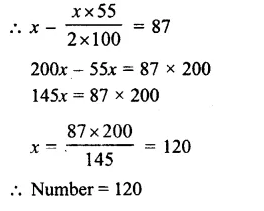## RS Aggarwal Class 8 Solutions Chapter 9 Percentage Ex 9B

These Solutions are part of RS Aggarwal Solutions Class 8. Here we have given RS Aggarwal Solutions Class 8 Chapter 9 Percentage Ex 9B.

Other Exercises

Tick the correct answer in each of the following:

Question 1.
Solution:
$$\\ \frac { 3 }{ 5 }$$
= $$\\ \frac { 3X20 }{ 5X20 }$$
= $$\\ \frac { 60 }{ 100 }$$
= 60% (d)

Question 2.
Solution:
0.8%
= $$\\ \frac { 0.8 }{ 100 }$$
= $$\\ \frac { 8 }{ 10X100 }$$
= $$\\ \frac { 8 }{ 1000 }$$
= 0.008 (b)

Question 3.
Solution:
6 : 5
= $$\\ \frac { 6 }{ 5 }$$
= $$\\ \frac { 6X20 }{ 5X20 }$$
= $$\\ \frac { 120 }{ 100 }$$
= 120% (c)

Question 4.
Solution:
5% of a number is 9
Number = $$\\ \frac { 9X100 }{ 5 }$$
= 180 (d)

Question 5.
Solution:
Let x% of 90 = 120
=> $$\\ \frac { x }{ 100 }$$ x 120 = 90
=> x% = $$\\ \frac { 120X100 }{ 90 }$$
= $$133\frac { 1 }{ 3 } %$$ (c)

Question 6.
Solution:
Let x% 10 kg = 250 g
$$\\ \frac { x }{ 100 }$$ x 10 kg
= $$\\ \frac { 250 }{ 1000 }$$ kg
x = $$\\ \frac { 250X100 }{ 1000X10 }$$
= $$\\ \frac { 25 }{ 10 }$$%
= 2.5% (d)

Question 7.
Solution:
40% of x = 240
=> x = $$\\ \frac { 240 }{ 40 }$$ x 100
= 600 (b)

Question 8.
Solution:
?% of 400 = 60
=> x% of 400 = 60
$$\\ \frac { x }{ 100 }$$ x 400 = 60
x = $$\\ \frac { 60X100 }{ 400 }$$
= 15 (c)

Question 9.
Solution:
(180% of ?)÷2 = 504
$$\left( \frac { 180 }{ 100 } \times x \right) \div 2$$ = 504
$$\frac { 180 }{ 100 } x$$ = 504 x 2
$$x=\frac { 504\times 2\times 100 }{ 180 }$$
= 560 (d)

Question 10.
Solution:
20% of Rs. 800
= $$\\ \frac { 20 }{ 100 }$$ x 800
= Rs 160 (a)

Question 11.
Solution:
Nitin gets = 98 marks
and it is 56% of total marks
Total-marks = $$\\ \frac { 98X100 }{ 56 }$$
= 175 (c)

Question 12.
Solution:
Let a number be = 1000
Then increase = 10%
Increased number = $$\\ \frac { 100X110 }{ 100 }$$ = 110
Now decrease = 1%
Decreased number = $$\\ \frac { 110X90 }{ 100 }$$ = 99
Difference = 100 – 99 = 1
% decrease = 1% (b)

Question 13.
Solution:
4 hours 30 min = $$4\frac { 1 }{ 2 } %$$
= $$\\ \frac { 9 }{ 2 }$$ hours
% of a day = $$\\ \frac { 9 }{ 2 }$$ x $$\\ \frac { 100 }{ 24 }$$%
= $$\\ \frac { 75 }{ 4 }$$%
= $$18\frac { 3 }{ 4 } %$$ (a)

Question 14.
Solution:
Let total number of examinees = 100
Passed = 65
Failed = 100 – 65 = 35
Now 35% of total examinees = 420
Total examinees = $$\\ \frac { 420X100 }{ 35 }$$
= 1200 (c)

Question 15.
Solution:
Let number = x
Then x = $$\\ \frac { xX20 }{ 100 }$$ = 40
=> 100x – 200x = 4000
80x = 4000
=> x = $$\\ \frac { 4000 }{ 80 }$$ = 50
Number = 50 (a)

Question 16.
Solution:
Rate of decrease = $$27\frac { 1 }{ 2 } %$$
Let number = xQuestion 17.
Solution:
Let x% of 20 = 0.05
$$\\ \frac { x }{ 100 }$$ x 20 = 0.05
x = $$\\ \frac { 0.05X100 }{ 20 }$$
= 0.25% (c)

Question 18.
Solution:
$$\\ \frac { 1 }{ 3 }$$ = 1206 = 402Question 19.
Solution:
x% of y is y% = $$\\ \frac { xy }{ 100 }$$
$$\\ \frac { y }{ 100 }$$ × x
= y% of x (a)

Question 20.
Solution:
Let x% of $$\\ \frac { 2 }{ 7 }$$ = $$\\ \frac { 1 }{ 35 }$$
=>$$\\ \frac { x }{ 100 }$$ x $$\\ \frac { 2 }{ 7 }$$ = $$\\ \frac { 1 }{ 35 }$$
x = $$\\ \frac { 1X100X7 }{ 35X2 }$$
= 10% (b)

Hope given RS Aggarwal Solutions Class 8 Chapter 9 Percentage Ex 9B are helpful to complete your math homework.

If you have any doubts, please comment below. Learn Insta try to provide online math tutoring for you.